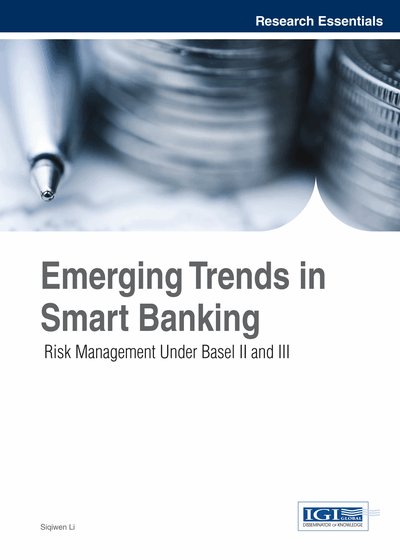# Coherent Risk Measures

DOI: 10.4018/978-1-4666-5950-6.ch007
Available
\$29.50
No Current Special Offers

## Abstract

This chapter introduces some alternative risk measures to Vale-At-Risk (VaR) calculations: Extreme Value Theory (EVT), Expected Shortfall (ES) and distortion risk measure. It also discusses their more coherent characteristics useful for shoring up the weaknesses of VaR.
Chapter Preview
Top

## Extreme Value Theory (Evt)

As discussed before, VaR methods tend to ignore extreme events at the tails of the probability distribution. One response to this problem is to use stress tests and scenario analysis. These can simulate the changes in the value of our portfolio under hypothesized extreme market conditions, and so can be very helpful. However, they are inevitably limited in that we cannot explore all possible scenarios. They also give us no indication of the likelihoods of the scenarios considered. Researchers and practitioners handle this issue by using Extreme Value Theory (EVT)—a specialized branch of statistics that attempts to make the best possible use of what little information we have about the extremes of the distributions in which we are interested.

Extreme value theory (EVT)1 models extreme values rather than whole sets of observations. As with VaR, its goal is to choose the height of the dike so as to balance the cost of construction against the expected cost of a catastrophic situation. EVT extends the central limit theorem2, which deals with the distribution of the average of the independent and identically distributed (i.i.d) random variables drawn from an unknown distribution to the distribution of their tails.

Fisher and Tippett (1928) proved the EVT theorem, which specifies the shape of the cumulative distribution function (cdf) for the value x beyond a cutoff point u. Under general conditions, the cdf belongs to:F(y) = 1- (1- ξy)-1/ ξ ξ≠0F(y) = 1- exp (-y) ξ =0where y=(x-u)/β, with β> 0 a scale parameter3. The parameter, ξ gives an indication of the heaviness of the tails: the bigger ξ, the heavier the tail. This parameter is known as the tail index, and the case of most interest in finance is where ξ >0, which corresponds to the fat tails commonly found in financial return data4. This theorem assumes the limiting distribution of extreme returns always has the same form (whatever the distribution of the parent returns from which extreme returns are drawn). It allows estimating extreme probabilities and extreme quantile, including VaR, without having to make strong assumptions about an unknown parent distribution.

This distribution is defined as the generalized Pareto distribution5 because it subsumes other known distributions, including the Pareto and normal distributions as special cases. The normal distribution corresponds to ξ=0, in which case the tails disappear at an exponential speed6. And heavy-tailed distributions do not necessarily have a complete set of moments, unlike the normal distribution. Indeed, E (Xk) is infinite to k≥ 1/ ξ7. Longin (1996) presents various methods to do so, we can then estimate the tail cdf as:(y)= 1- (Nu/ N).

## Complete Chapter List

Search this Book:
Reset